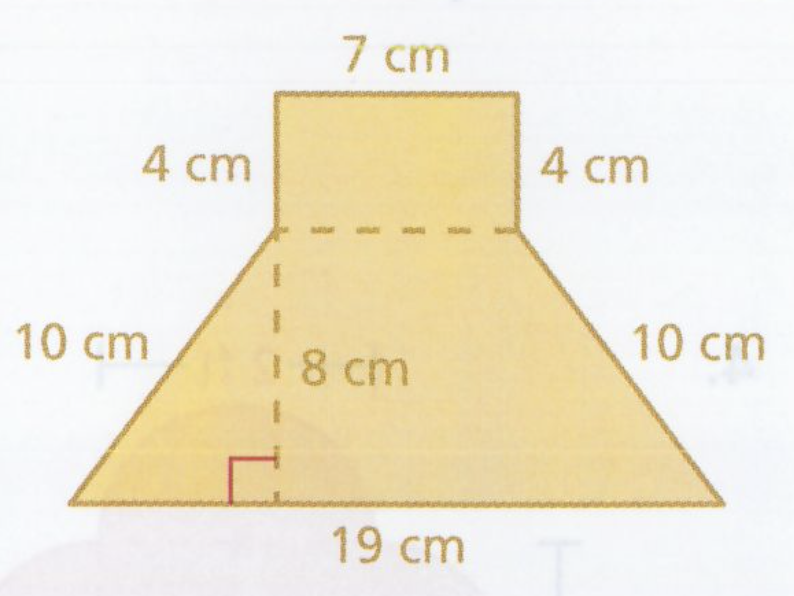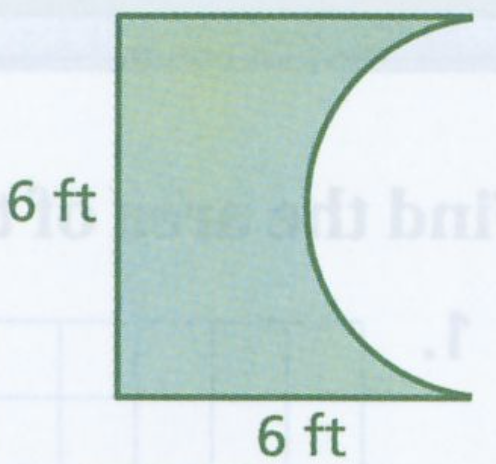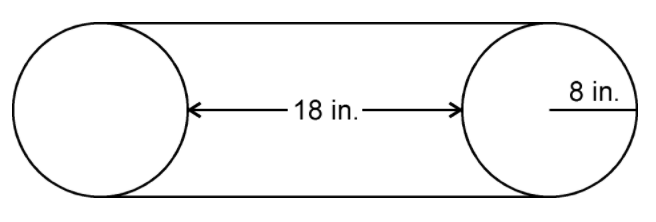Areas of Composite Figures
starstarstarstarstarstarstarstarstarstar
by Felix Gabathuler
| 22 Questions
1
1
Which number is 0.02% of 50?
0.01
0.1
1
1002
1
A composite shape is the same thing as a compound figure. In the space below, draw a composite shape with includes parts of an obtuse triangle, a semi-circle, and a square.
3
1
You have recently discovered how to solve for the area of a circle and quadrilateral. If you need a refresher course on finding the area of a triangle, click on the following lesson. It'll only take a minute... really. If you feel good about your triangle skills, skip it and move on.

Area of Triangles
Completed/skipped it
Neither complete nor skipped... I'm confused.
If you'd like to watch a few videos on finding the areas of composite shapes, click on one or more below. If you feel comfortable with your skills or you just want to jump into the fire, move on to the questions!
4
1
Look at the image below. Show how you would cut the composite shape into more manageable pieces whose areas are easily calculated. There are many correct answers.
5
1
What is the area (in square units) of the green figure in Question 4? Do not include units.
6
1
Look at the image below. Show how you would cut the composite shape into more manageable pieces whose areas are easily calculated. There are many correct answers.
7
1
What is the area (in square units) of the blue figure in Question 6? Do not include units.
8
1
Below you see a trapezoid. Could that technically be considered a composite shape? Cut up the shape and find the area of each piece. Write the area of the whole trapezoid in the space below the figure.
9
1
If you think you need a little more practice finding the area of compound shapes, try to the following lesson:

Area of Composite Shapes

If you think you're ready for some more challenging problems, start on the next question. Good luck!
Finished/Didn't need practice
Tried but didn't understand
Watch the video below about how to find the area of a trapezoid. It might prove useful... very useful... to you in the future!10
1
Find the area of the figure above. Do not include units.11
1
Find the area of the figure above. Round your answer to the nearest tenth.12
1
Find the area of the figure above to the nearest tenth. Do not include units.13
1
Professional pizza ovens are often lined with stone or brick. This is so heat is stored up and redistributed evenly. To get a similar result at home, pizza stones can be used. Pizza stones are made of the same material that lines blast furnaces and kilns so they can withstand ultra-high temperatures.

An 8-inch diameter pizza stone costs \$14.35. Marco estimated that a 16-inch diameter pizza stone (same thickness) should cost \$28.70. Based on the areas of the two pizza stones, is Marco’s estimate reasonable? Explain your answer.14
1
Calculate the area (in square cm) of the shaded portion in the image above. Round your answer to the nearest hundredth. Do not include units.15
1
Well that was weird... But anyway, complete the following lesson as Part 1 of the Gator Challenge.

Area Challenge
That gator is no match for me!
I'm missing my left arm... not good.16
1
Give the design for two different kinds of envelopes above, which one has the greater area? Write left or right.
17
1
Using the same picture above, you make 500 envelopes using the design with the greater area. Using the same amount of paper, how many more envelopes can you make with the other design?18
1
A mechanic needs to replace the belt around two wheels, as shown above. The radius of each wheel is 8 inches. What is the length of the belt, in inches? Round to the nearest hundredth and do not include units.
19
1
Using the same image as Question 18, if the same belt were put on two wheels with a 12 inch diameter, approximately how far apart would the wheels have to be? Round to the nearest tenth and do not include units.20
1
A wire is bent into the shape of a rectangle with a width of 7 and a length of 12.

The wire is then bent into a different shaped rectangle which is not a square. Sketch such a rectangle below and find the values for its length and width. Show and explain how you found these numbers.
21
1
A wire is bent into the shape of a rectangle with a width of 7 and a length of 12.

The wire is then bent into a square. Sketch the square and determine the length of one of its sides. Show and explain how you found this number.
22
1
A wire is bent into the shape of a rectangle with a width of 7 and a length of 12.

The wire is then bent into a circle. Sketch the circle and determine the length of its diameter. Show and explain how you found this number.